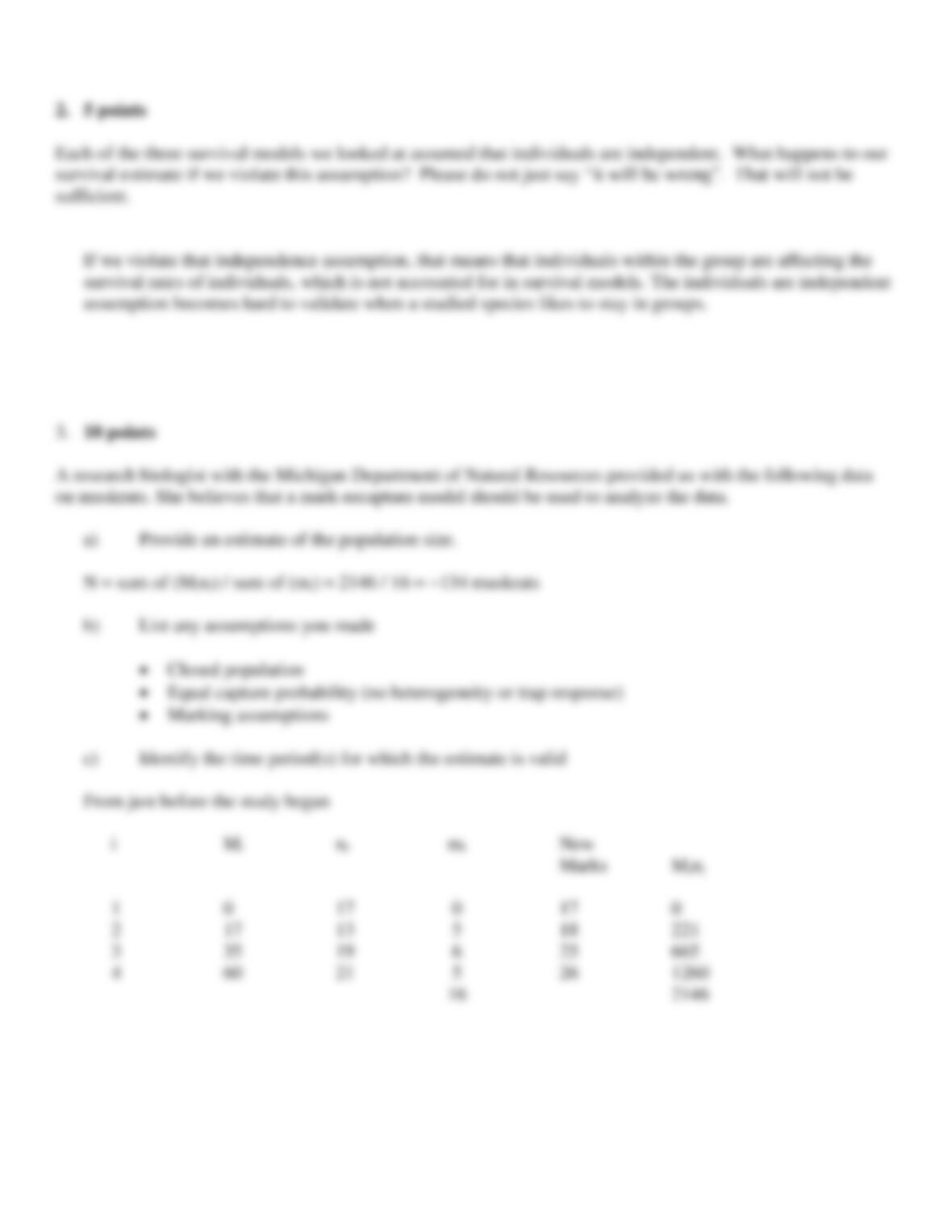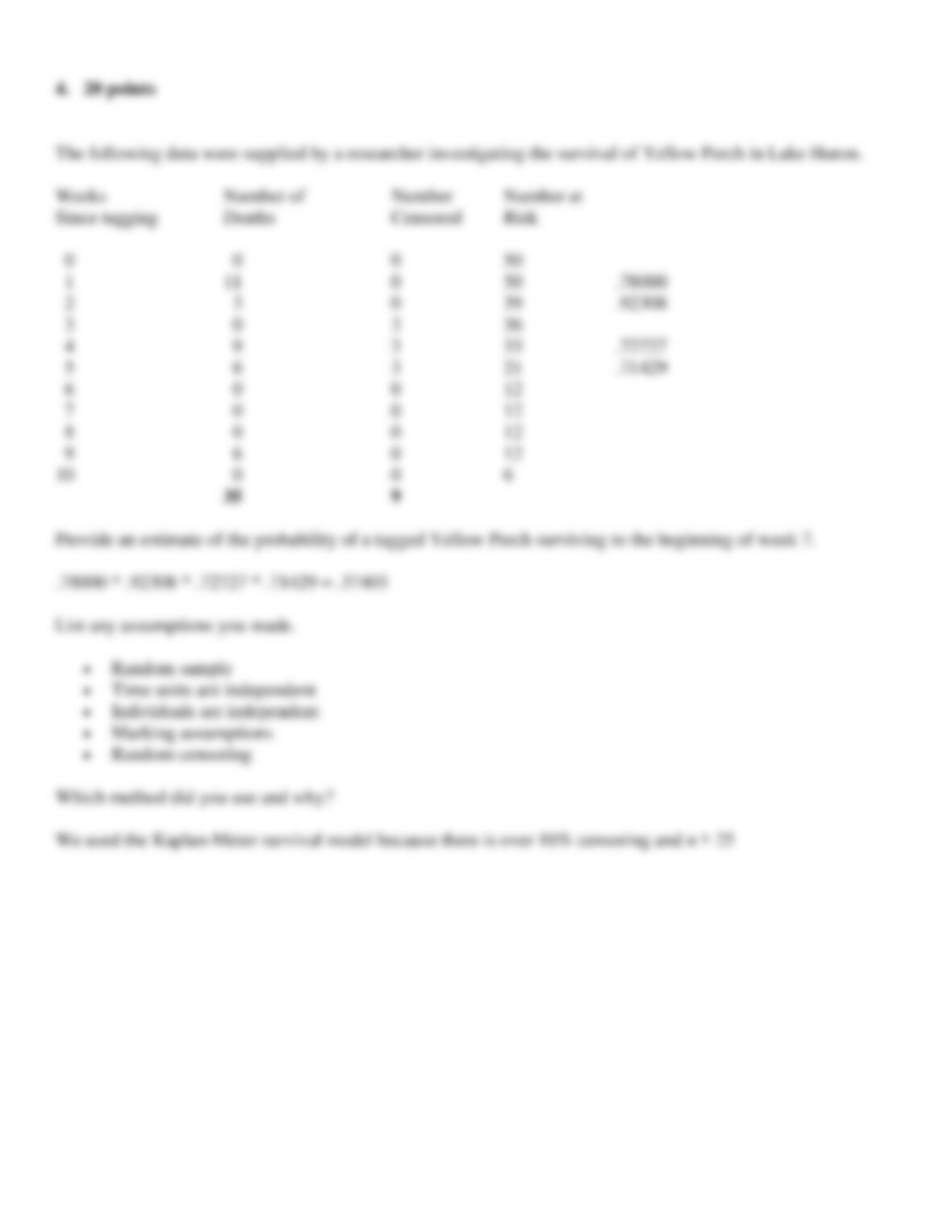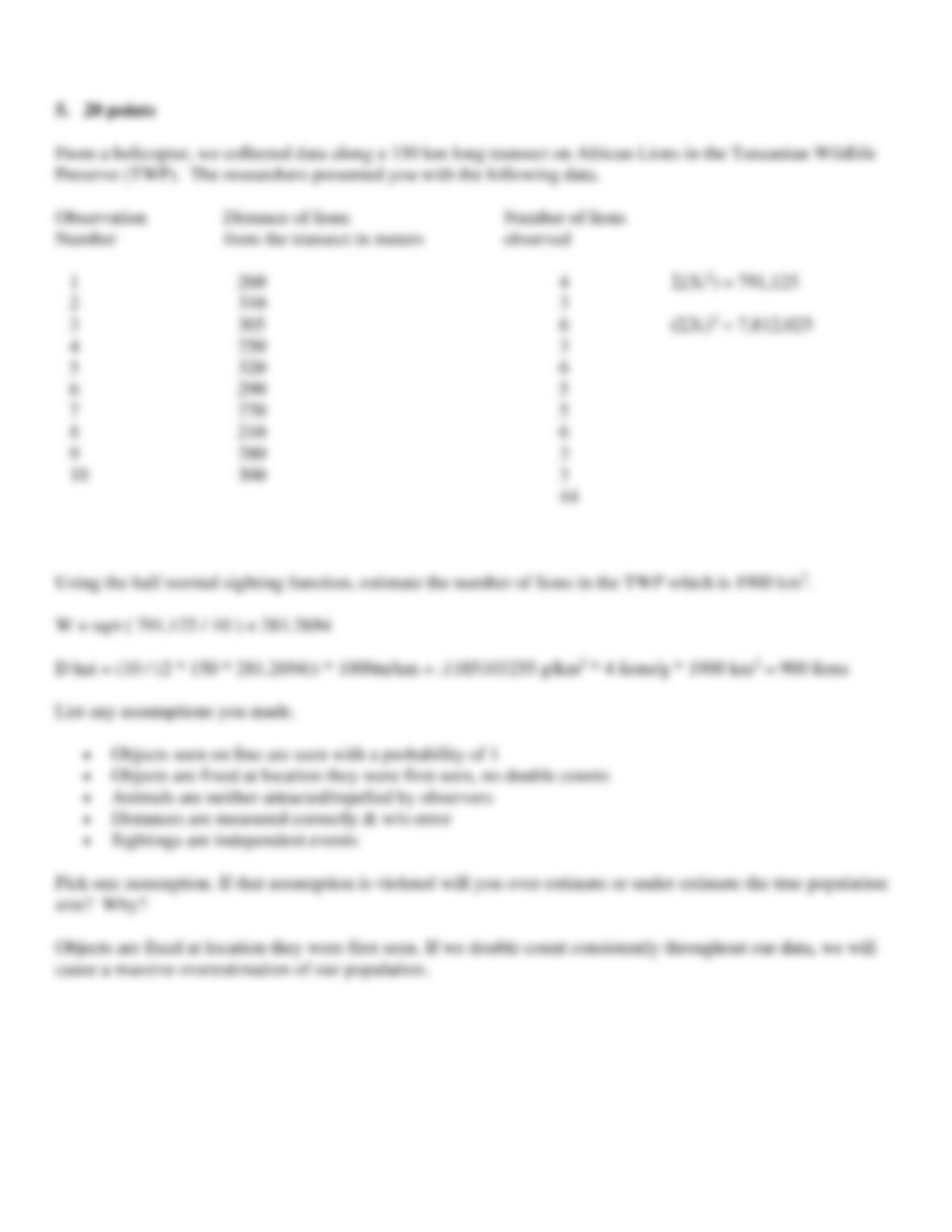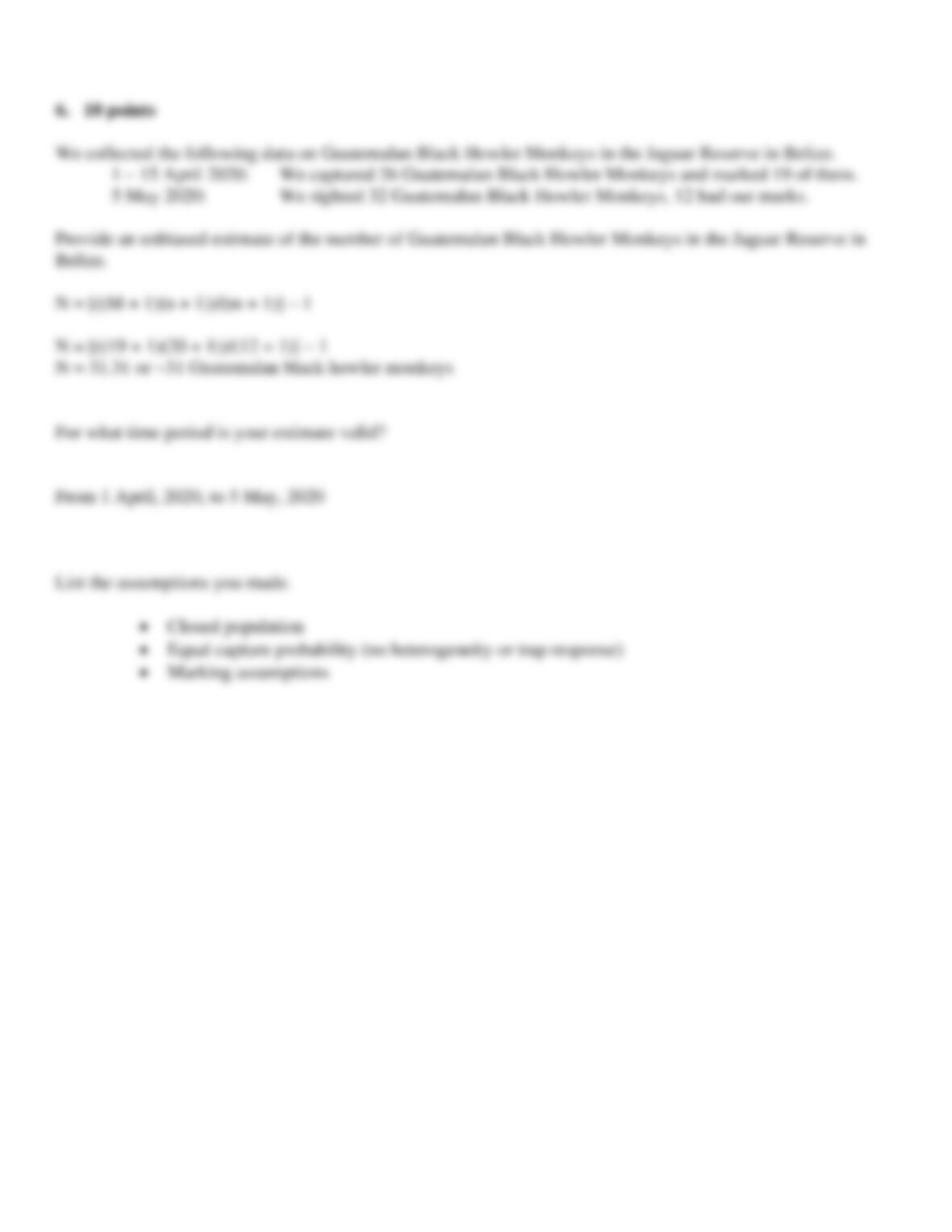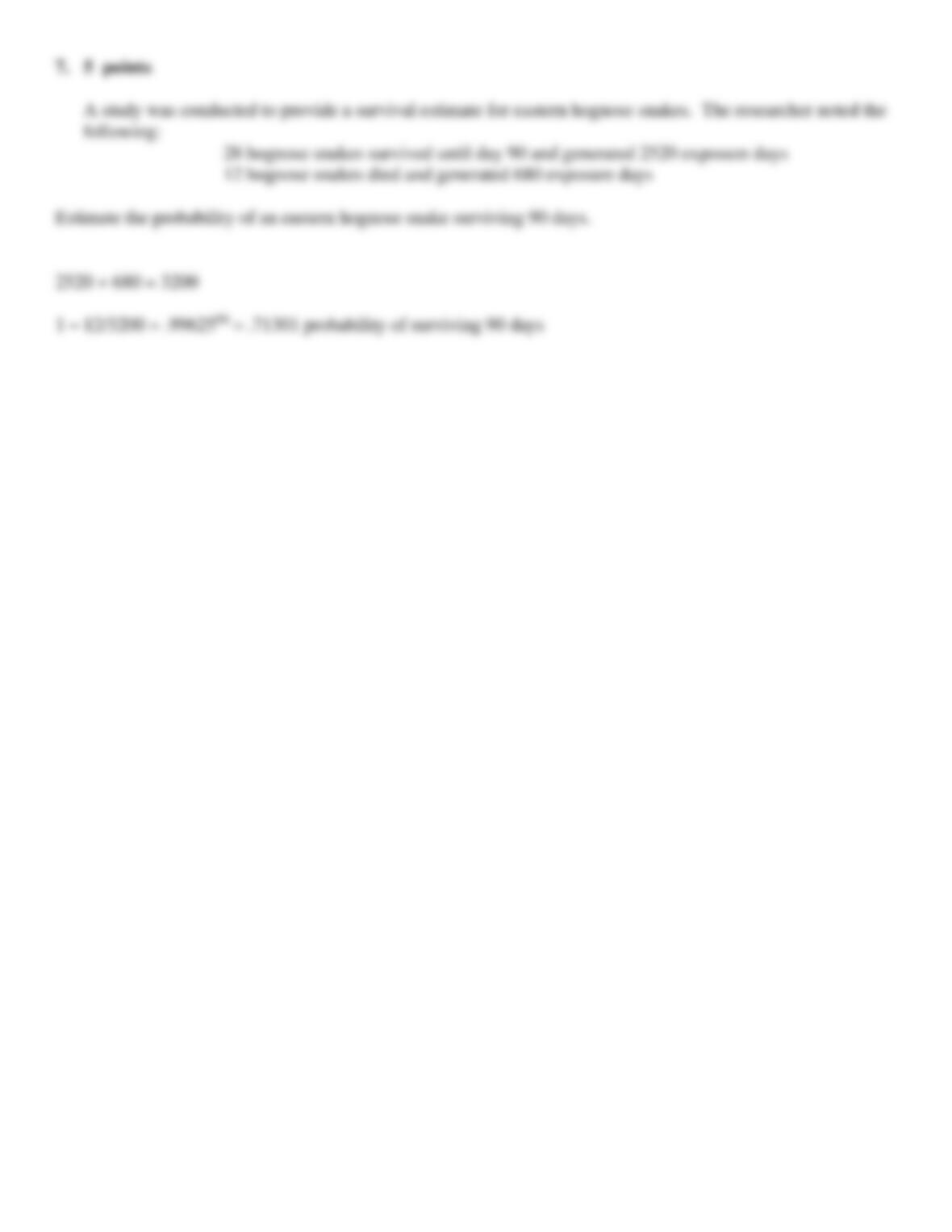Type
Essay
Pages
7 pages
Word Count
1059 words
School
Michigan State Universit
Course Code
FW424

November 20, 2020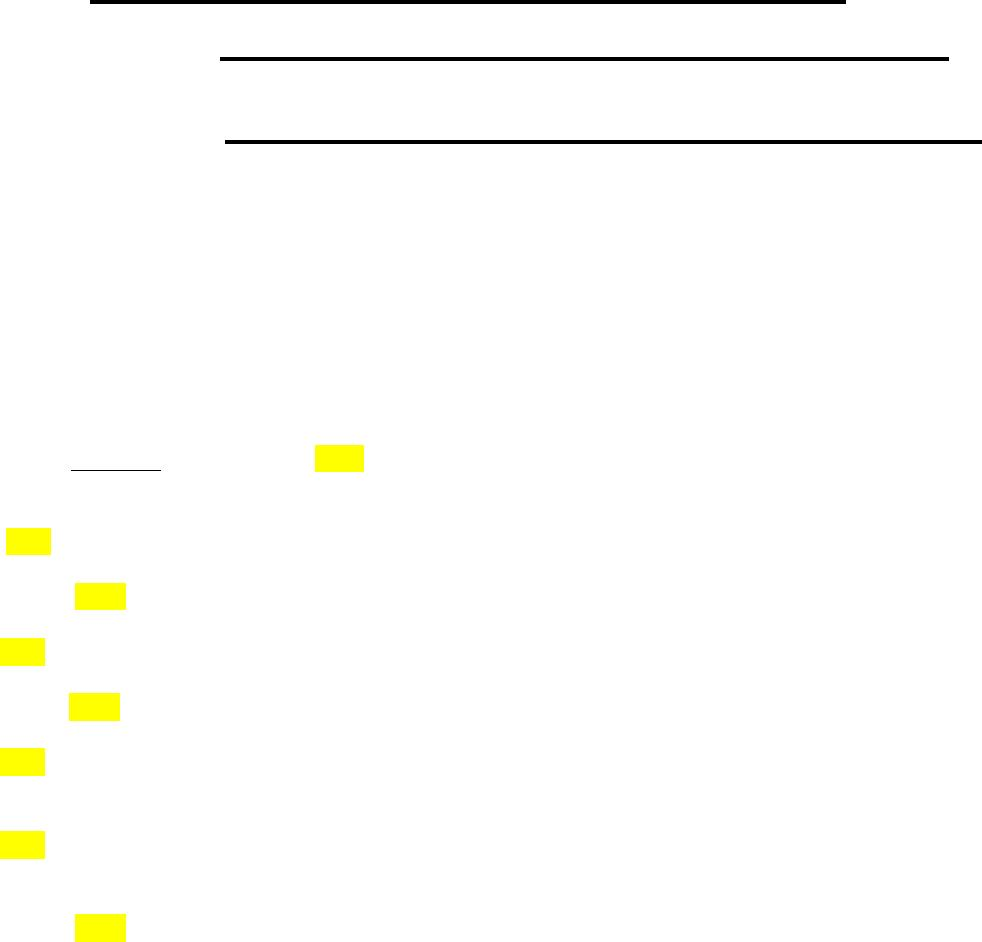FW424 MIDTERM EXAMINATION #2 Fall 2020
NAME Marshall Weimer
START DATE & TIME 3:00 11/13/20
END DATE & TIME 4:45 11/13/20
This examination is worth 100 points. You have a maximum of two hours to complete the exam. Be concise
but be thorough. Partial credit may be given for problems requiring calculations, so show your work. The
exam is open book. You can use any class-related materials. Do not use search engines. This exam is a strictly
1. 30 points total (A G: 2 points each; H K: 4 points each)
(For the True or False and multiple choice questions make sure that your answer is obvious.
Circle, underline, highlight with color or highlight with Bold.)
A. True False In a life table analysis, lx can be used to determine if an animal is r or K selected.
B. True False In an occupancy study, ψ will tell us which sites are occupied.
C. True False px = 0 for the oldest age class listed in a life table.
D. True False In a sightability model, the correction factor can be less than 1.0.
E. True False When applied to the same data, using a uniform sighting function can never result in a
lower density estimate when compared to using an exponential sighting function.
F. True False If I use the Mayfield method to analyze survival data, because I assume constant survival,
all of the animals in my study must have the same to.
G. True False Since populations can decrease in size, even though it is called the finite rate of increase,
λ can be negative.
H. For what values of λ can a population be at Stable Age Distribution?
λ can be any value, since stable age distribution does not tell us what is happening to the growth rate of the
population.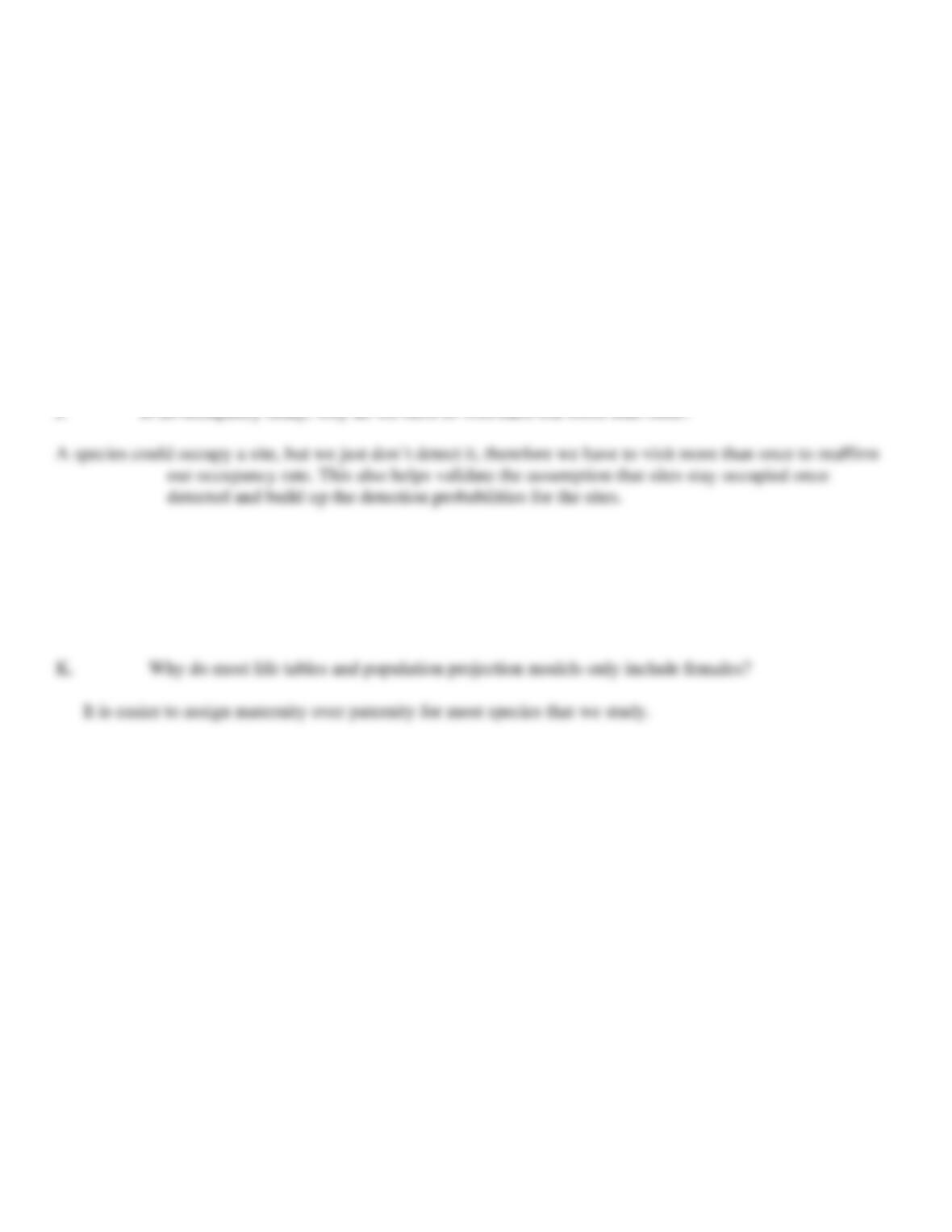I. In a sightability study, when we are collecting the data to build the model to get our correction
factors, why is it necessary to send the crew in the airplane to one or two areas that we know do not have
Since we are developing a model for estimates, not correction factors, we have to fly over areas without radioed
animals so we can know the true size of the population. We have to try and simulate what would happen if we
tried for real to get an estimate of the population size.